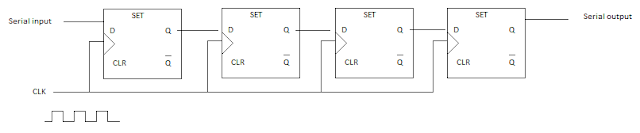A register is a type of digital circuit. It is made by using some Flip-Flops. A limited number of bit can be saved in the register.

## Register

In the microprocessors normally in data processing, the data are saved for a little time. That's why the registers are called "Temporary memory".

Each Flip-Flop in a register can save only a single binary bit(either 0 or 1). So, In n bit registers n number of Flip-Flops are exists. These can contain binary information of n bit.

With Flip-Flop there is also a Combinational gate for creating the path of feedback or different types of data processing system building.

Overall it can be said it is a circuit build by Flip-Flops which can save binary information.

####### Uses

• In microprocessor: For saving temporary data.
• Saving information: Giving command of different Low-level programming language information can be saved in the register. As a cash memory, it plays an important role.

####### Types

2. Shift register

The registers which can contain or load the data is called load register. It is also called a buffer register.

#### Shift  register:

Containing which registers can also shift the data right or left is called the shift register.
Considering the working procedures we can find two types of registers:

1. Normal registers
2. Special registers

#### Normal registers:

These types of registers can be used in all the working procedures.

#### Special registers:

In any special purpose or specific problem solving different types of special registers are used.

Example- Accumulator register, program counter register, memory address register, data register, signal register, buffer register.
The parallel load registers which load multiple numbers of binary bits and also can give the output is called parallel load register.

The Block diagram of a simple parallel load register block diagram is given below-

4 bit parallel register structured by 4 flip-flops 4 D type. Here are a common clear line and a common clock pulse.

It can save binary information of 4 bits. When a common clock pulse is given in the input then the input of I₀, I₁, I₂, and I₃ binary bit transfers into the binary bit register. It stays before not giving any inputs. This saved 4-bit data can be get from output X₀, X₁, X₂, and X₃. Here we have to give 1 as input for clear. If we give 0 as input for clear it will reset the data.

An example: Let, I₀=0, I₁=1, I₂=1 and I₃=0 and the CLK Puls =1

then output X₀=0,X₁=1,X₂= 1 and X₃=0.

#### Serial in a serial-out shift register

In shift registers a bundle of Flip-Flops is connected like a chain so, the output from a single Flip-Flop keeps connection with the next Flip-Flop. A common clock pulse is got in all Flip-Flops which updates as the condition changes.

The simplest serial in the serial-out shift register figure is given below where only D type Flip-Flop has been used. The output from Flip-Flop is connected with the input of the next Flip-Flop. A common clock pulse has given. In a single pulse, one bit is transferred.Structure of a Simple serial in the serial-out shift register

Different types of shift registers-

1. Shift left
2. Shift right
3. Controlled shift
Controlled shift registers have two types. They are-

In a 4 bit shift right register we can save a number like this.

In this case, 4 D type Flip-Flops are used.

Let, Q₃,Q₂,Q₁,Q₀ = 0000 and later Q₃,Q₂,Q₁,Q₀ = 1101 will be saved.

Here,
• 1st clock pulse where the value of CP 0 to 1 then Q₃, Q₂, Q₁, Q₀= 1000
• 2nd clock pulse where the value of CP 0 to 1 then Q₃, Q₂, Q₁, Q₀= 1001
• 3rd clock pulse where the value of CP 0 to 1 then Q₃, Q₂, Q₁, Q₀= 1010
• 4th clock pulse where the value of CP 0 to 1 then Q₃, Q₂, Q₁, Q₀= 1011

In a 4 bit shift left register we can save a number like this.

Here 4 D type Flip-Flop has been used. Each output is connected with the input and there is a common clock pulse.

If primary Q₃,Q₂,Q₁,Q₀ = 0000 and later Q₃,Q₂,Q₁,Q₀ = 1101 will be loaded. While CP = 1 bit is shifted and till the CP = 0 it continues.

Here,

1st clock pulse where the value of CP becomes 0 to 1 then Q₃, Q₂, Q₁, Q₀= 1000

2nd clock pulse where the value of CP becomes 0 to 1 then Q₃, Q₂, Q₁, Q₀= 1001

3rd clock pulse where the value of CP becomes 0 to 1 then Q₃, Q₂, Q₁, Q₀= 1010

4th clock pulse where the value of CP becomes 0 to 1 then Q₃, Q₂, Q₁, Q₀= 1011